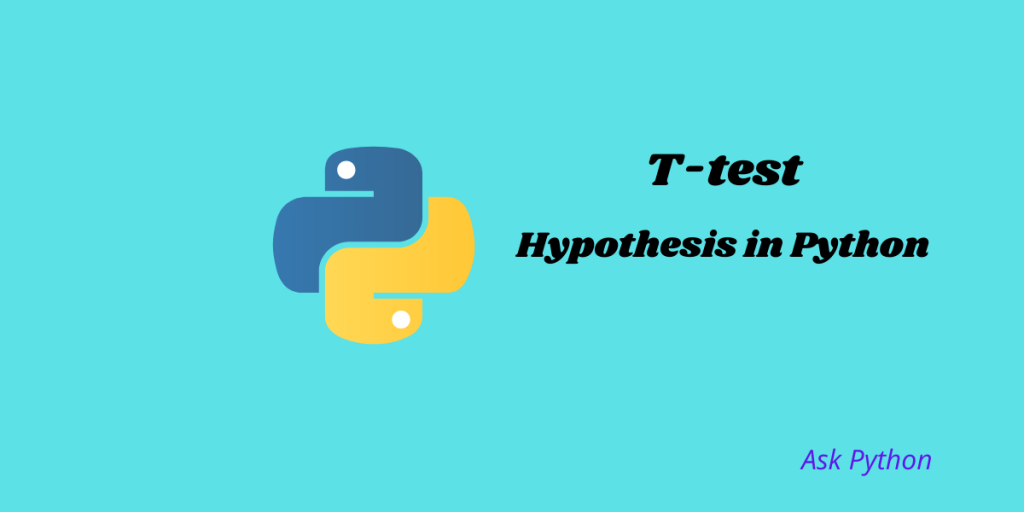# T-Test Hypothesis in PythonThere are various types of statistical tests available for every kind of study, whether it is a biological study or a population study. The Student’s T-test, or simply T-test, is another such test that is used for assessing the mean between two different groups. It is called the two-sample T-test, or with a particular value, which is called the one-sample T-test.

In this article, we will discuss the T-test in detail. Let’s get started!

## What Is a T-Test and Why Is It Useful?

A T-test is a parametric test that is used to draw inferences after comparing means for different groups or with a specific mean for a specific group. T-test follows the t-distribution which is a type of continuous probability distribution.

T-tests are specifically useful for small sample size data(n<=30), unlike Z-tests which are only useful for large sample data. Even for the very small size of data(n<=5) t-tests are very useful.

## What Is p-Value and Alpha?

P-value actually gives the measure of the probability that you will get a larger value than the value you obtained by doing an experiment. Alpha gives the probability of rejecting the null hypothesis when actually it is true. The value of alpha is 5 % or 0.05. So if the p-value is greater than alpha we will accept the null hypothesis and if it is lesser than alpha we will accept the alternative hypothesis.

## Types of T-test

There are 3 types of T-Tests that you can work with. Let’s look at each of them in detail and learn how to implement them in Python.

### 1. One-Sample T-test

One-sample t-test or single sample t-test is used to compare the mean of a random sample of a population with the mean of the population that is already known. For example, we know that the average birth weight for babies in India is 2,499 grams and now we want to compare the average birth weight of a sample of babies to this already known mean value.

Now let’s take a look at the hypotheses for this test.

• Null Hypothesis: In this case, the sample mean is equal to the known mean value of the population.
• Alternative Hypothesis: In this case, the sample mean can be greater or lesser than the known population mean.
• Another alternative hypothesis can be the sample mean which is not equal to the known mean value of the population.

Let’s have a look at how we can implement this in Python.

• Null hypothesis: Mean of the areas is 5000.
• Alternative hypothesis: Mean of the areas is not  5000.
```import scipy.stats as stats
import pandas as pd

t_statistic, p_value = stats.ttest_1samp(a=data, popmean=5000)
print(t_statistic , p_value)
```

Output:

```[-0.79248301] [0.44346471]
```

Here we can see the p-value is greater than 0.05 and hence we will accept the null hypothesis and reject alternative hypothesis.

### 2. Two-sample t-test

Two sample t-tests or unpaired to independent test is used to determine how two individual groups differ from each other by comparing their means. In this type of t-test, first, the sample means are derived, and then from the sample means, the population means or the unknown means are derived.

For example: There are two groups and we want to compare how significantly different these two groups are by comparing their mean values.

Note: The two groups should be sampled independently from the same population.

Let’s look at the hypotheses for this type of t-test.

• Null Hypothesis: It is true when two group means are equal.two
• Alternative Hypothesis: When two group means are different.

Let’s see how to interpret this in python.

```import numpy as np

group1 = np.array([14, 15, 15, 16, 13, 8, 14, 17, 16, 14, 19, 20, 21, 15, 15, 16, 16, 13, 14, 12])
group2 = np.array([15, 17, 14, 17, 14, 8, 12, 19, 19, 14, 17, 22, 24, 16, 13, 16, 13, 18, 15, 13])
import scipy.stats as stats

stats.ttest_ind(a=group1, b=group2, equal_var=True)
```

Output:

```Ttest_indResult(statistic=-0.6337397070250238, pvalue=0.5300471010405257)
```

Since p-value is greater than 0.05 we will accept the null hypothesis.

### 3. Paired T-test

Paired t-test is used to compare the difference between a pair of dependent variables for the same subject or population. You can use this type of t-test specifically when you have paired measurements. You can apply this test before and after measurement.

For example, You can examine the effect of a medication on a population. You can see the effect of medicine before and after medication.

Let’s take a look at the hypotheses.

• Null Hypothesis: Both the dependent variables are equal and the difference between them is equal to zero.
• Alternative Hypothesis: There is a difference between the two dependent variables.
• Another alternative hypothesis can be that the two independent variables can be either greater or lesser than zero.

Note: The observations for this type of test should be sampled independently of each other.

Let’s see how we can implement this in Python. In this case, we will generate data on pre-medication and post-medication in a population.

```pre = [88, 82, 84, 93, 75, 79, 84, 87, 95, 91, 83, 89, 77, 90, 91]
post = [91, 84, 88, 90, 79, 80, 88, 90, 90, 96, 88, 85, 81, 74, 92]
import scipy.stats as stats
stats.ttest_rel(pre, post)
```

Output:

```Ttest_relResult(statistic=-0.36856465236305264, pvalue=0.7179658269802107)
```

The two-sided p-value is greater than 0.05 and thus we accept the null hypothesis.

## Conclusion

In this article, we learned about different t-tests in Python. The t-test has the advantage that it can be applied to a very small sample size. In the case of paired t-test, it is better that the two groups have a high-within pair correlation or r greater than 0.8 if the sample size is small.